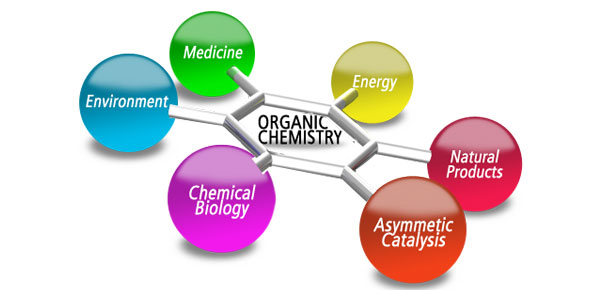# Mixed Quiz On Nomenclature

26 Questions | Attempts: 223
ShareSettingsMixed Nomenclature: Honors ChemistryMonatomic and Polyatomic Ions, Ionic Compounds, Acids, Hydrocarbons, and Binary Covalent Compounds*Remember: Acids are ionic, and hydrocarbons are covalent!

• 1.
When written in words, transition metals require roman numerals.
• A.

True

• B.

False

• 2.
Which of the following are true?
• A.

Arsenate has more oxygen atoms than sulfate.

• B.

Arsenate has a greater negative charge than sulfate.

• C.

When arsenate and aluminum bond, the chemical formula is AlAsO4.

• D.

Nitride and arsenate have the same charge.

• 3.
Polyatomic ions with the prefix "bi" tend to have hydrogen atoms.
• A.

True

• B.

False

• 4.
Which of the following are true?
• A.

Monatomic anions are usually gases.

• B.

Ionic metals tend to end with the suffix -ide.

• C.

Since ammonium's atoms are all gaseous, it is a binary covalent compound.

• D.

Almost all ionic compounds have metals

• 5.
Which of the following lists correctly orders the anions from least amount of oxygen atoms to most?
• A.

Oxide, Acetate, Oxalate, Tartrate

• B.

Bromite, Hypobromite, Perbromate, Bromate

• C.

Hypochlorite, Chlorite, Perchlorate, Chlorate

• D.

Thiocyanate, Hypochlorite, Arsenate, Dichromate

• 6.
Fill in the blanks (even though the first blank is a Roman numeral, just write the number): The formula for zirconium (___) hypochlorite is Zr(ClO)___.
• 7.
HIO4 is the name of the compound ________.
• 8.
The formula of __________ is S2O3 2-.
• 9.
The formula for non___ is C9H16
• A.

Ane

• B.

Ene

• C.

Yne

• D.

None of the above

• 10.
Group ___ elements like oxygen form ions with a charge of -2.
• 11.
Group 13 elements like aluminum form ions with a charge of ___.
• 12.
Name the compound: Sb2S5
• 13.
Name the compound: NH4C2H3O2
• 14.
Write the formula for mercury (I). Use a space to separate the charge from the rest of the formula.
• 15.
Write the formula of the covalent compound that is not a hydrocarbon: lithium hydroxide; diphosphorus pentoxide; octyne
• 16.
Write the formula or name (based on which one it is) of the compound that is not an acid: H2Te; sodium tartrate
• 17.
Write the name of the ionic compound: Cu2S; PCl3
• 18.
P4O6 is the formula for what compound?
• 19.
What is the name of the compound with the formula Cr(MnO4)6?
• 20.
What is the name of the compound with the formula CF4?
• 21.
What is the chemical formula of silver phosphide?
• 22.
Write the formula of calcium arsenite.
• 23.
Write the name of the ionic compound: P4O10; HCN
• 24.
The formula for hypobromous acid is...

## Related TopicsBack to top
×

Wait!
Here's an interesting quiz for you.